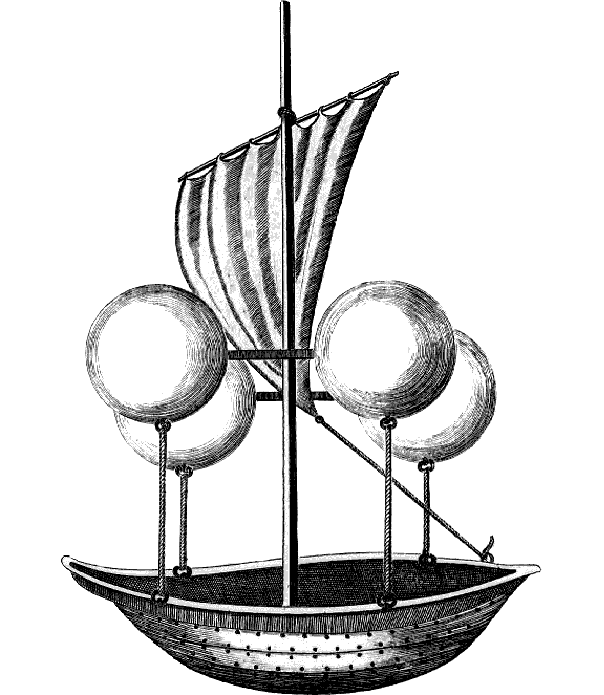The Vacuum Airship

Archimedes’ Principle states that the (upthrust) force on an object that is displacing a fluid is equal to the weight of the fluid displaced. For example, a cube with sides of one metre, fully submerged in water, will experience an upward force of 9810 newtons, as this is the weight of one cubic metre of water. The material that the object is made of has no effect on this force, so if the object weighs more than 9810 newtons it will sink, and if it weighs less than 9810 newtons it will float.

The upthrust force on an object therefore depends only on the relative densities of the object and the fluid it is displacing. A bigger difference means a bigger force.

Hydrogen is the least dense gas, at 0.0898 kilograms per metre cubed, but hydrogen is rarely used in airships as it is highly flammable and therefore dangerous. Hydrogen was used in the Hindenburg because  helium was difficult to produce and the United States, the only country with significant reserves, had banned its export. Helium has a density of 0.179 kg/m3 and therefore produces only 93% of the lift of hydrogen, but it is far, far safer.Ideally, to create the maximum upthrust force, we would want our balloon or airship’s envelope to be filled with something with the lowest possible density. The lowest possible density would be a vacuum, the total absence of anything, which would create a lifting force of 12.7 newtons per cubic metre (as opposed to 11.0 N/m3 for helium).

The problem with using a vacuum to lift an airship, is how to contain the vacuum. If a difference in pressure exists between two regions, then a difference in force exists between those two regions. In the case of a vacuum airship being used on Earth’s surface, that force would be 101325 newtons per square metre, the equivalent of more than ten tonnes pushing down on every square metre. No material on Earth is strong enough to withstand this force without being so heavy as to negate the point of the vacuum lifting effect in the first place. In order to still have lifting capability and withstand the stresses involved, we can calculate the minimum required ratio of Young’s modulus to density, and this yields a figure of around 450000 Pa/(kg/m3)2. Unfortunately, even the strongest materials, like diamond, have ratios that are only one-fifth of this, so it looks like we won’t be creating vacuum airships any time soon.

3 thoughts on “The Vacuum Airship”

1. According to our finite element analysis, it is possible to fabricate a vacuum airship using currently available materials (please see our patent application 11/517915 at the USPTO site or at http://akhmeteli.org/wp-content/uploads/2011/08/vacuum_balloons_cip.pdf ). The calculation you refer to relates to a homogeneous spherical shell, whereas one can use a sandwich shell, thus dramatically increasing stability with respect to buckling.

2. Venter says:

On theoretical transportation, is the physics of the displacement field drive (kickstarter) totally sound? Would there said relativistic fields be sufficient.

3. It looks ridiculous. I doubt it very much.